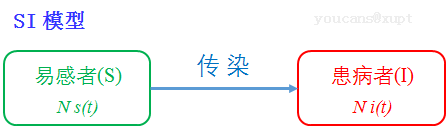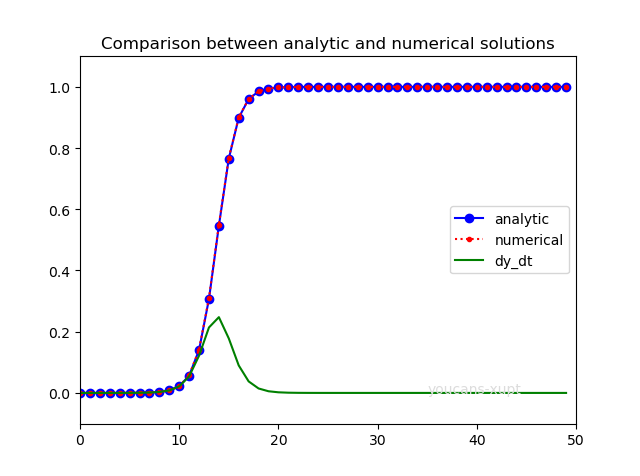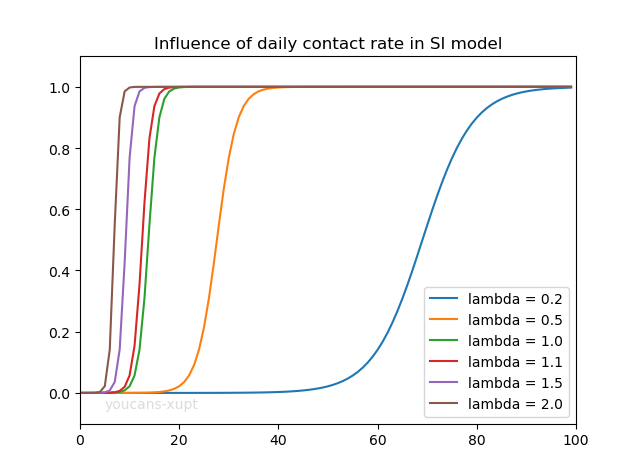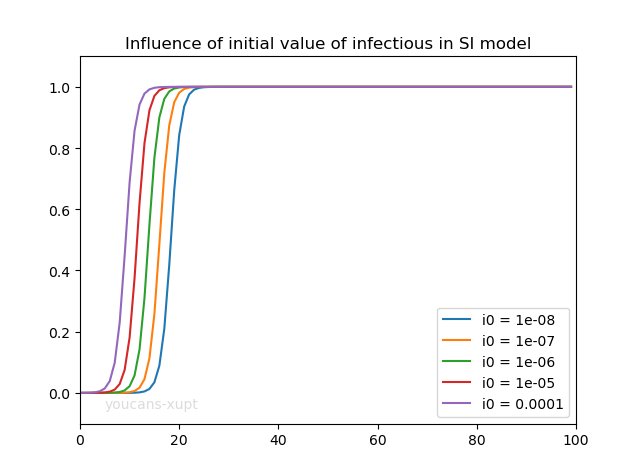# Python小白的数学建模课-B2. 新冠疫情 SI模型

SI 模型是最简单的传染病模型，适用于只有易感者和患病者两类人群。

『Python小白的数学建模课 @ Youcans』带你从数模小白成为国赛达人。

## 1. 前言

• S 类（Susceptible），易感者，指缺乏免疫能力的健康人，与感染者接触后容易受到感染；

• E 类（Exposed），暴露者，指接触过感染者但暂无传染性的人，适用于存在潜伏期的传染病；

• I 类（Infectious），患病者，指具有传染性的患病者，可以传播给 S 类成员将其变为 E 类或 I 类成员；

• R 类（Recovered），康复者，指病愈后具有免疫力的人。如果免疫期有限，仍可以重新变为 S 类成员，进而被感染；如果是终身免疫，则不能再变为 S类、E类或 I 类成员。

## 2. 疫情传播 SI 模型

### 2.1 SI 模型的适用范围

SI 模型适用于只有易感者和患病者两类人群，且无法治愈的疾病，例如 T型病、僵尸。### 2.2 SI 模型的假设

1. 考察地区的总人数 N 不变，即不考虑生死或迁移；
2. 人群分为易感者（S类）和患病者（I类）两类；
3. 易感者（S类）与患病者（I类）有效接触即被感染，变为患病者，无潜伏期、无治愈情况、无免疫力；
4. 每个患病者每天有效接触的易感者的平均人数（日接触数）是 $\lambda$，称为日接触率；
5. 将第 t 天时 S类、I 类人群的占比记为 $s(t)$$i(t)$，数量为 $S(t)$$I(t)$；初始日期 $t=0$ 时， S类、I 类人群占比的初值为 $s_0$$i_0$

### 2.3 SI 模型的微分方程

\begin{align*} N\frac{di}{dt} = N\lambda s i \end{align*}

\begin{align*} \frac{di}{dt} = \lambda i (1-i)，\ i(0) = i_0 \end{align*}

\begin{align*} & i(t)=\frac{1}{1+(1/i_0 - 1)\ e^{-\lambda t}}\\ & I(t)= N\ i(t) \end{align*}

## 3. SI 模型的 Python 编程

### 3.2 SI 模型的数值解

SI 模型是常微分方程初值问题，可以使用 Scipy 工具包的 scipy.integrate.odeint() 函数求数值解，具体方法可以参考前文《Python小白的数学建模课-09 微分方程模型》。

scipy.integrate.odeint(func, y0, t, args=())


**scipy.integrate.odeint() **是求解微分方程的具体方法，通过数值积分来求解常微分方程组。

odeint() 的主要参数：

• func: callable(y, t, …) 　　导数函数 $f(y,t)$ ，即 y 在 t 处的导数，以函数的形式表示
• y0: array：　　初始条件 $y_0$，对于常微分方程组 $y_0$ 则为数组向量
• t: array：　　求解函数值对应的时间点的序列。序列的第一个元素是与初始条件 $y_0$ 对应的初始时间 $t_0$；时间序列必须是单调递增或单调递减的，允许重复值。
• args: 向导数函数 func 传递参数。当导数函数 $f(y,t,p1,p2,..)$ 包括可变参数 p1,p2.. 时，通过 args =(p1,p2,..) 可以将参数p1,p2.. 传递给导数函数 func。

odeint() 的返回值：

• y: array 　　数组，形状为 (len(t),len(y0)，给出时间序列 t 中每个时刻的 y 值。

odeint() 的编程步骤：

1. 导入 scipy、numpy、matplotlib 包；
2. 定义导数函数 $f(i,t)=\lambda i (1-i)$
3. 定义初值 $i_0$$i$ 的定义区间 $[t_0,\ t]$
4. 调用 odeint() 求 $i$ 在定义区间 $[t_0,\ t]$ 的数值解。

### 3.3 Python例程：SI 模型的解析解与数值解

# 1. SI 模型，常微分非常，解析解与数值解的比较
from scipy.integrate import odeint  # 导入 scipy.integrate 模块
import numpy as np  # 导入 numpy包
import matplotlib.pyplot as plt  # 导入 matplotlib包

def dy_dt(y, t, lamda, mu):  # 定义导数函数 f(y,t)
dy_dt = lamda*y*(1-y)  # di/dt = lamda*i*(1-i)
return dy_dt

# 设置模型参数
number = 1e7  # 总人数
lamda = 1.0  # 日接触率, 患病者每天有效接触的易感者的平均人数
mu1 = 0.5  # 日治愈率, 每天被治愈的患病者人数占患病者总数的比例
y0 = i0 = 1e-6  # 患病者比例的初值
tEnd = 50  # 预测日期长度
t = np.arange(0.0,tEnd,1)  # (start,stop,step)

yAnaly = 1/(1+(1/i0-1)*np.exp(-lamda*t))  # 微分方程的解析解
yInteg = odeint(dy_dt, y0, t, args=(lamda,mu1))  # 求解微分方程初值问题
yDeriv = lamda * yInteg *(1-yInteg)

# 绘图
plt.plot(t, yAnaly, '-ob', label='analytic')
plt.plot(t, yInteg, ':.r', label='numerical')
plt.plot(t, yDeriv, '-g', label='dy_dt')
plt.title("Comparison between analytic and numerical solutions")
plt.legend(loc='right')
plt.axis([0, 50, -0.1, 1.1])
plt.show()


### 3.4 解析解与数值解的比较## 4. SI 模型参数的影响

### 4.1 日接触率对 SI 模型的影响1. 日接触率越大，疫情从发生到爆发的时间越短，爆发过程的增长速度也越快。
2. 不论日接触率多大，患病者的比例最终都会增长到 1，表明所有人都被感染成为患者。
3. 不论日接触率多大，都具有缓慢发展、爆发、增长放缓 3 个阶段，进入爆发阶段后患病者的比例急剧增长，疫情就很难控制了。

### 4.2 患病者比例的初值对 SI 模型的影响### 4.3 SI 模型结果讨论

1. $i(t)=0.5,\ I(t) = N/2$ 时 $di/dt$ 达到最大值，病人数目 $I(t)$ 增加最快。由此可以预报传染病高潮的到来，即为医院的门诊量最大的一天，卫生部门要重点关注。
2. $t_m$$\lambda$ 成反比。日接触率 $\lambda$ 反映卫生水平、防控手段，提高卫生水平、强化防控手段，降低病人的日接触率，可以推迟传染病高潮的到来。
3. 当 $t \to \infty$ 时 $i \to 1$ ，表明所有人最终都会被传染而变成病人。这完全不符合实际情况，表明该模型太不讲 politics 了，只能适用于美帝国家建模。
4. SI 模型非常明显而严重的缺陷，是该模型没有考虑患病者可以治愈，因此只能是健康人患病，而患病者不能恢复健康（甚至也不会死亡，而是不断传播疫情），所以终将全部被传染。

【本节完】